• 数字图像的加噪去噪高斯噪声、椒盐噪声，均值滤波、中值滤波、维纳滤波。
• matlab开发-超越高斯去噪剩余学习深度信息去噪。超越高斯去噪：用于图像去噪的深CNN残差学习
• 采用MATLAB，对三幅经典的图像分别加上高斯噪声、乘性噪声、椒盐噪声，并分别使用均值滤波、中值滤波、高斯低通滤波、巴特沃斯滤波、PCA、小波变换、DCT等去噪方法对其进行处理。去除噪声方法效果以PSNR（峰值信噪比...
• 在医学图像处理、遥感图像处理、图像修复等图像处理中，总希望能得到...本文以Matlab语言为基础用各种方法对图像进行去噪处理，并且对于处理结果进行比较、分析，从而得出各自的特点以备在图像处理中可以方便的选择。
• 本文主要研究分析邻域平均法中值滤波法维纳滤波法及模糊小波变换法的图像去噪算法首先介绍图像处理应用时的常用函数及其用法其次详细阐述了四种去噪算法原理及特点最后运用Matlab软件对一含噪图片含高斯噪声或椒盐...
• 实验二 图像去噪 一实验目的 熟悉图像高斯噪声和椒盐噪声的特点 掌握利用均值滤波和中值滤波去除图像噪声的方法 二实验内容 打开 Matlab 编程环境 读入图像在图像上分别添加高斯噪声和椒盐噪声 显示原图像和噪声图像...
• 该文档提供了利用matlab自带函数实现高斯滤波的方法。
• 2、添加不同强度的高斯噪声、椒盐噪声，编程实现图像的均值滤波、高斯加权均值滤波、中值滤波运算，对比分析不同尺寸平滑模板对高斯噪声和椒盐噪声的去噪效果差异。 1.1对图像添加椒盐噪声和高斯噪声 通过imnoise...
实验内容
1.自选一幅图像或自制一幅图像，图像的主体目标物形状有块状、圆形等形状，且有细线及尖角细节等多样化的细节。
2、添加不同强度的高斯噪声、椒盐噪声，编程实现图像的均值滤波、高斯加权均值滤波、中值滤波运算，对比分析不同尺寸平滑模板对高斯噪声和椒盐噪声的去噪效果差异。
1.1对图像添加椒盐噪声和高斯噪声
通过imnoise函数分别对图像添加椒盐噪声和高斯噪声，即I2=imnoise(I1,‘gaussian’,0,0.04);
P2=imnoise(I1,‘salt & pepper’,0.1);
当添加方差为0.04的高斯噪声和噪声密度为0.1的椒盐噪声后，图像分别变为如下图所示：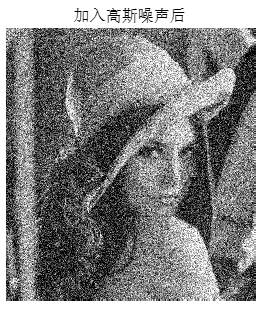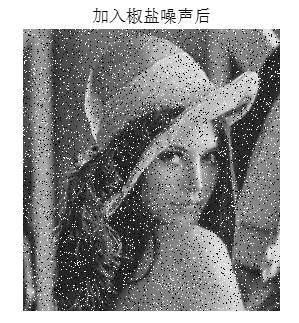1.2图像去噪
分别通过均值滤波模板和高斯加权均值滤波模板对图像的像素点进行挨个处理，得到去噪后的图像，在使用中值滤波时对图像使用sort()函数进行排序，取中间的像素点作为该位置的像素点灰度值，处理后的图像如下图所示：
当高斯噪声方差为0.04时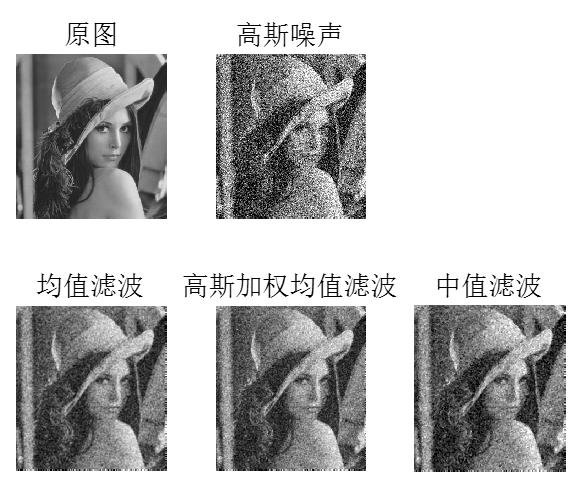由图像结果可以看出，加入高斯噪声后图像变得不平滑，而在分别进行均值滤波，高斯加权均值滤波和中值滤波后，图像变得平滑，但同时清晰度也降低了。
当椒盐噪声密度为0.2时：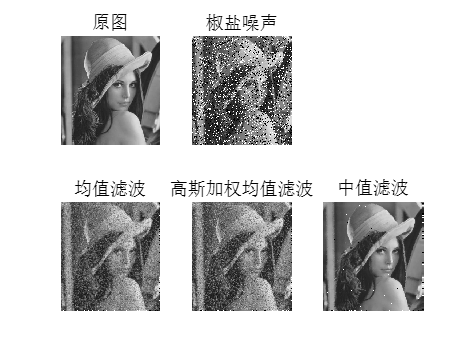可以看出中值滤波非常适合处理椒盐噪声。
附代码如下：
clc
clear all
close all
A1 = imread('lenna.jpg'); % 读入图像
A1=rgb2gray(A1);    %将彩色图转化为二维的灰度图
I1=im2double(A1);

V=0.12;
I2=imnoise(I1,'gaussian',0,V);%加入高斯噪声
P2=imnoise(I1,'salt & pepper',0.4); %加入椒盐躁声
figure;
imshow(I2),title('加入高斯噪声后');
figure;
imshow(P2),title('加入椒盐噪声后');
[row,col]=size(I2);   %获取图像的像素点个数
I3=I2;
P3=P2;
for i=2:row-1
for j=2:col-1
I3(i,j)=(I2(i,j)+I2(i-1,j)+I2(i+1,j)+I2(i,j-1)+I2(i,j+1)+I2(i-1,j-1)+I2(i-1,j+1)+I2(i+1,j-1)+I2(i+1,j+1))/9;
P3(i,j)=(P2(i,j)+P2(i-1,j)+P2(i+1,j)+P2(i,j-1)+P2(i,j+1)+P2(i-1,j-1)+P2(i-1,j+1)+P2(i+1,j-1)+P2(i+1,j+1))/9;
end
end

I4=I2;
P4=P2;
for i=2:row-1
for j=2:col-1
I4(i,j)=(I2(i,j)*4+I2(i-1,j)*2+I2(i+1,j)*2+I2(i,j-1)*2+I2(i,j+1)*2+I2(i-1,j-1)+I2(i-1,j+1)+I2(i+1,j-1)+I2(i+1,j+1))/16;
P4(i,j)=(P2(i,j)*4+P2(i-1,j)*2+P2(i+1,j)*2+P2(i,j-1)*2+P2(i,j+1)*2+P2(i-1,j-1)+P2(i-1,j+1)+P2(i+1,j-1)+P2(i+1,j+1))/16;
end
end

a=zeros(1,9);
c=zeros(1,9);
I5=I2;
P5=P2;
for i=2:row-1
for j=2:col-1
a=[I2(i,j),I2(i-1,j),I2(i+1,j),I2(i,j-1),I2(i,j+1),I2(i-1,j-1),I2(i-1,j+1),I2(i+1,j-1),I2(i+1,j+1)];
c=[P2(i,j),P2(i-1,j),P2(i+1,j),P2(i,j-1),P2(i,j+1),P2(i-1,j-1),P2(i-1,j+1),P2(i+1,j-1),P2(i+1,j+1)];
b=sort(a);
d=sort(c);
I5(i,j)=b(5);
P5(i,j)=d(5);
end
end
figure;
subplot(2,3,1),imshow(I1),title('原图','fontsize',16);
subplot(2,3,2),imshow(I2),title('高斯噪声','fontsize',16);
subplot(2,3,4),imshow(I3),title('均值滤波','fontsize',16);
subplot(2,3,5),imshow(I4),title('高斯加权均值滤波','fontsize',16);
subplot(2,3,6),imshow(I5),title('中值滤波','fontsize',16);
figure;
subplot(2,3,1),imshow(I1),title('原图','fontsize',16);
subplot(2,3,2),imshow(P2),title('椒盐噪声','fontsize',16);
subplot(2,3,4),imshow(P3),title('均值滤波','fontsize',16);
subplot(2,3,5),imshow(P4),title('高斯加权均值滤波','fontsize',16);
subplot(2,3,6),imshow(P5),title('中值滤波','fontsize',16);

三种滤波方法的对比分析：
1、均值滤波是一种线性滤波器，处理思路也很简单，就是将一个窗口区域中的像素计算平均值，然后将窗口中计算得到的均值设置为锚点上的像素值。
该算法有优点在于效率高，思路简单。同样，缺点也很明显，计算均值会将图像中的边缘信息以及特征信息“模糊”掉，会丢失很多特征。
2、高斯滤波是一种线性滤波，是常用的一种滤波算法，利用二维高斯函数的分布方式来对图像进行平滑。
高斯滤波的优点可以集中在高斯函数的特点上来看
首先，二维高斯函数是旋转对称的，在各个方向上平滑程度相同，不会改变原图像的边缘走向。
第二，高斯函数是单值函数，高斯卷积核的锚点为极值，在所有方向上单调递减，锚点像素不会受到距离锚点较远的像素影响过大，保证了特征点和边缘的特性。
第三，在频域上，滤波过程中不会被高频信号污染。
3、中值滤波是一种非线性滤波，在处理脉冲噪声以及椒盐噪声时效果极佳，能够有效的保护好图像的边缘信息。
中值滤波的处理思路很简单，取卷积核当中所覆盖像素中的中值作为锚点的像素值即可。


展开全文• Step2：输入噪声系数 ➡ 选择加入噪声类型（例子：加入高斯噪声） Step3:选择去噪类型，完成去噪； 注意：如果想使用其它类型 噪声 和 去噪 方法，需要点击 清除 按钮，清除之操作内容，再从Step1进行操作。 ...
第一步：点击打开untitled.m文件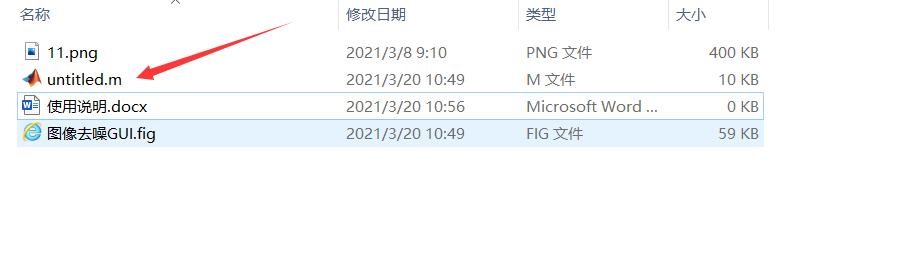第二步：点击运行文件，出现GUI操作页面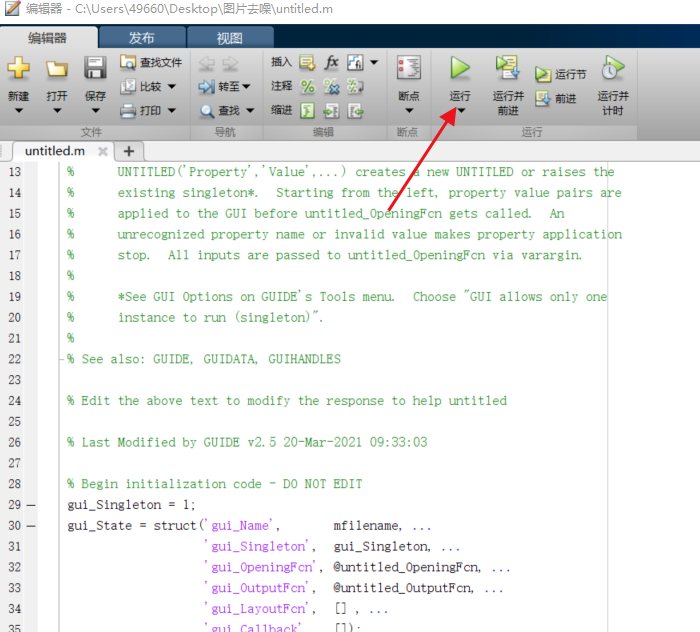第三步： GUI操作说明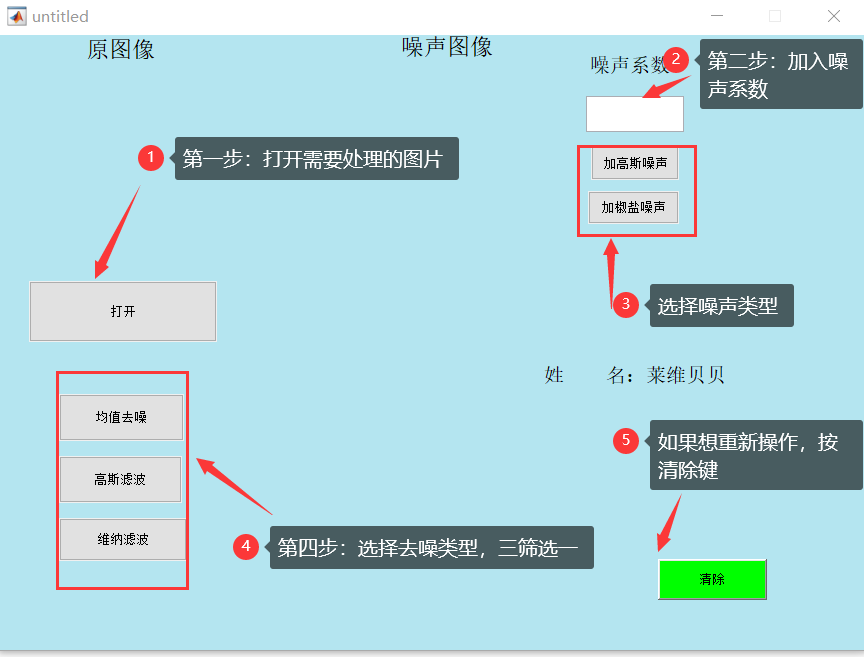Step1：打开处理的图片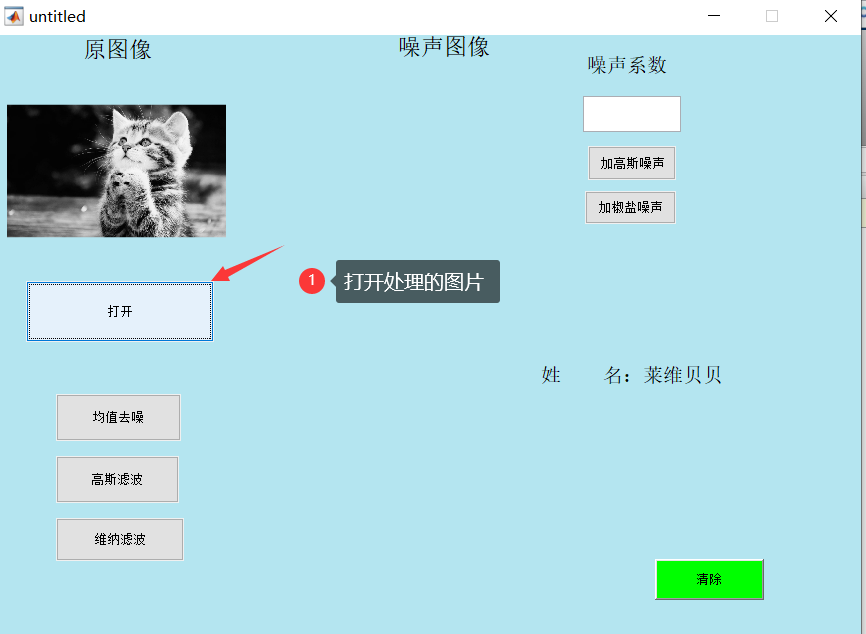Step2：输入噪声系数 ➡ 选择加入噪声类型（例子：加入高斯噪声）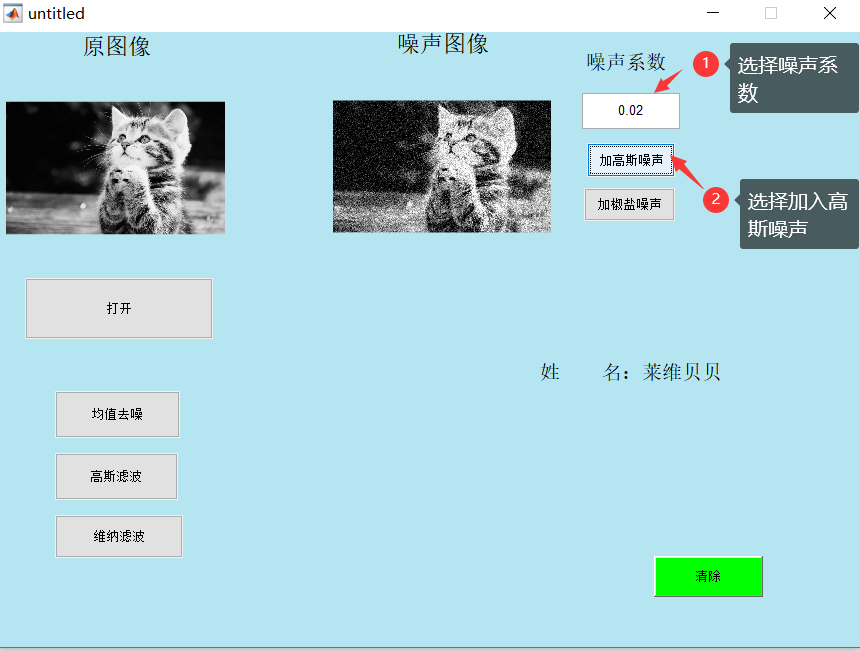Step3:选择去噪类型，完成去噪；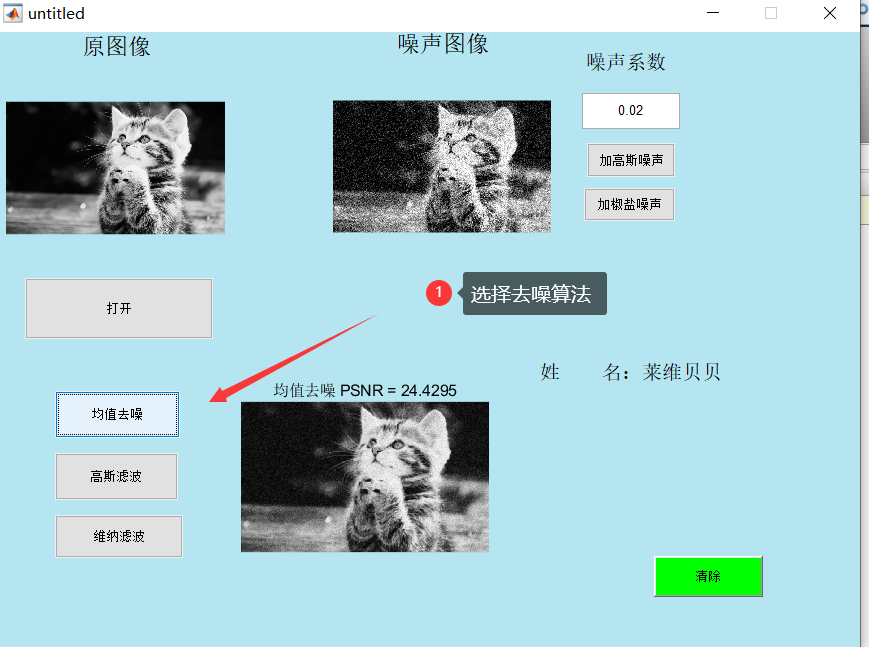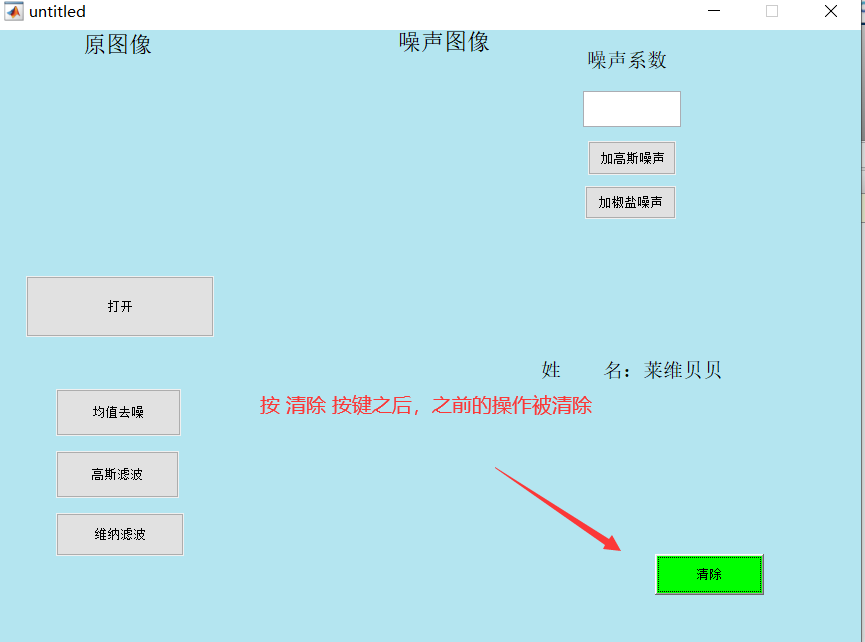注意：如果想使用其它类型 噪声 和 去噪 方法，需要点击 清除 按钮，清除之操作内容，再从Step1进行操作。

视频演示：https://www.bilibili.com/video/BV17i4y1A7zZ?pop_share=1

完整代码下载：https://download.csdn.net/download/A496608119/16633089?spm=1001.2014.3001.5501


展开全文• 实用文案 数字图像去噪典型算法及 matlab 实现 希望得到大家的指点和帮助 图像去噪是数字图像处理中的重要环节和步骤去噪效果的好坏直接影响到后续的图像处理工作如图像分割边缘检测等图像 信号在产生 传输过程中都...
• ## matlab图像去噪处理

热门讨论 2009-05-24 11:23:48
有关MATLAB编程下的图象去噪处理，均值滤波，中值滤波，Sigma平滑滤波器，K近邻(KNN)平滑滤波分别对高斯噪声图像和椒盐噪声图像进行噪声抑制。
• 综合分析了几种用于图像消噪的方法 ,并通过实验比较了各种消噪方法的效果 .实验表明 ,对于非线性高斯白噪声 ,小波分析方法优于中值滤波和自适应维纳滤波 ;加权中值滤波适宜于滤除脉冲噪声 ,而自适应维纳滤波对于滤除...
• ## Matlab图像去噪（中值和均值）

万次阅读 多人点赞 2017-12-21 14:41:51
图像信号在产生、传输过程中都可能会受到噪声的污染，一般数字图像系统中的常见噪声主要有：高斯噪声（主要由阻性元器件内部产生）、椒盐噪声（主要是图像切割引起的黑图像上的白点噪声或光电转换过程中产生的泊松...
简单概念
图像去噪是数字图像处理中的重要环节和步骤。去噪效果的好坏直接影响到后续的图像处理工作如图像分割、边缘检测等。图像信号在产生、传输过程中都可能会受到噪声的污染，一般数字图像系统中的常见噪声主要有：高斯噪声（主要由阻性元器件内部产生）、椒盐噪声（主要是图像切割引起的黑图像上的白点噪声或光电转换过程中产生的泊松噪声）等；这里用一个函数实现 ：imnoise函数。（见文末）
这里说明两种图像去噪算法：
均值滤波算法
也称线性滤波，主要思想为邻域平均法，即用几个像素灰度的平均值来代替每个像素的灰度。有效抑制加性噪声，但容易引起图像模糊，可以对其进行改进，主要避开对景物边缘的平滑处理。
中值滤波
基于排序统计理论的一种能有效抑制噪声的非线性平滑滤波信号处理技术。中值滤波的特点即是首先确定一个以某个像素为中心点的邻域，一般为方形邻域，也可以为圆形、十字形等等，然后将邻域中各像素的灰度值排序，取其中间值作为中心像素灰度的新值，这里领域被称为窗口，当窗口移动时，利用中值滤波可以对图像进行平滑处理。其算法简单，时间复杂度低，但其对点、线和尖顶多的图像不宜采用中值滤波。很容易自适应化。
话不多说附代码：

I=imread('C:\Users\wpc\Pictures\1.jpg');%读取图像

I = rgb2gray(I);%灰度处理

J=imnoise(I,'salt & pepper',0.2);%加入椒盐噪声，密度为0.2

subplot(2,3,1);imshow(I);

title('原始图像');

subplot(2,3,2); imshow(J);

title('加入椒盐噪声之后的图像');

%采用MATLAB中的函数filter2对受噪声干扰的图像进行均值滤波

K1= medfilt2(J);%采用二维中值滤波函数medfilt2对受椒盐噪声干扰的图像滤波

%采用MATLAB中的函数filter2对受噪声干扰的图像进行中值滤波

K2=filter2(fspecial('average',3),J)/255; %模板尺寸为3

K3=filter2(fspecial('average',5),J)/255;% 模板尺寸为5

K4= filter2(fspecial('average',9),J)/255; %模板尺寸为9

subplot(2,3,3);imshow(K1);

title('中值滤波');

subplot(2,3,4); imshow(K2);

title('均值滤波，尺寸3');

subplot(2,3,5);imshow(K3);

title('均值滤波，尺寸5');

subplot(2,3,6);imshow(K4);

title('均值滤波，尺寸9');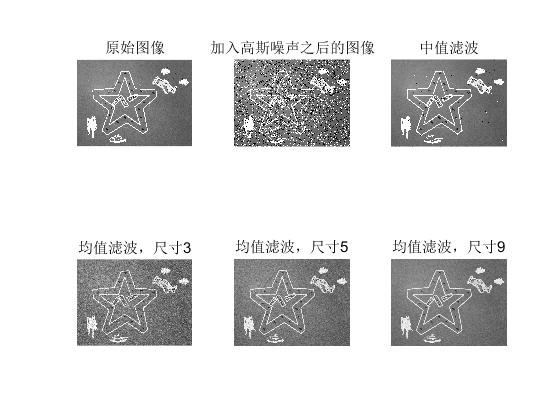分析与总结
通过目测?
中值滤波效果要比均值好
使用均值滤波去噪效果选用的邻域半径越大效果越好，当然其代价也会更大。
PS:
fspecial函数
fspecial函数用于创建预定义的滤波算子，其语法格式为：
h = fspecial(type)
h = fspecial(type,parameters)


参数type制定算子类型，parameters指定相应的参数，具体格式为：
type=‘average’，为均值滤波，参数为n，代表模版尺寸，用向量表示，默认值为[3,3]。

imnoise函数
imnoise（I,type）


I：为处理的后的图像矩阵type：添加噪声的类型，包含 ‘gaussian’ 、‘salt & pepper’…（不会自己查。。。啦啦啦）


展开全文函数
• matlab图像去噪 选择lena作为测试，添加高斯白噪声进行去噪验证
• 该工具包中包含有一些很常用的图像处理算法，如图像高斯平滑、各项同性高斯平滑、向异性高斯平滑、全变分去噪、加窗傅里叶变换等
• 一.应用背景在信息化的社会里，图像在信息传播中所起的作用越来越大。所以，消除在图像采集和传输过程中而产生的噪声，保证图像受污染度...最后运用Matlab软件对一张含噪图片（含高斯噪声或椒盐噪声）进行仿真去噪...
一.应用背景在信息化的社会里，图像在信息传播中所起的作用越来越大。所以，消除在图像采集和传输过程中而产生的噪声，保证图像受污染度最小，成了数字图像处理领域里的重要部分。二、研究内容本文主要研究分析邻域平均法、中值滤波法及小波变换法的图像去噪算法。首先介绍图像处理应用时的常用函数及其用法；其次详细阐述了三种去噪算法原理及特点；最后运用Matlab软件对一张含噪图片（含高斯噪声或椒盐噪声）进行仿真去噪，通过分析仿真结果得出：均值滤波是典型的线性滤波，对高斯噪声抑制是比较好的；中值滤波是常用的非线性滤波方法，对椒盐噪声特别有效；对小波系数进行阈值处理可以在小波变换域中去除低幅值的噪声和不期望的信号。三、目录推荐摘 要	IAbstract	II目 录	III第1章 概 述	11.1 课题研究背景	11.2 图像去噪的研究现状	11.3 本文主要工作	2第2章 图像去噪算法	52.1 图像噪声概述	52.2 邻域平均法图像去噪	62.3 中值滤波法图像去噪	72.4 基于小波变换法图像去噪	112.5 本章小结	17第3章 基于Matlab的图像去噪算法仿真	183.1 邻域平均法的仿真	183.2 中值滤波的仿真	203.3 基于小波变换法的仿真	223.4 几种去噪方法的比较分析	253.5 本章小结	26第4章 GUI系统的设计	274.1 GUI界面介绍	274.2 GUI界面设计	274.3 运行效果	29参考文献	36致 谢	37四、GUI界面设计4.1 GUI界面介绍图形用户接口GUI是用户和计算机程序之间进行信息交流的方式。通过图形用户界面，用户不需要输入脚本或命令，不需要了解任务的内部运行方式，计算机在屏幕显示图形和文本，若有扬声器还可产生声音。用户通过输入设备，键盘，鼠标，麦克风等与计算机进行通信。图形用户界面GUI中包含多个图形对象，如图标，菜单，文本的用户界面。以某种方式选择或激活这些对象，引起相应的动作或变化，最常用的激活方式是用鼠标控制屏幕上的鼠标指针运动。图形用户界面GUI具有操作方便，控制灵活的特点，已成为现代应用程序的主要方式。4.2 GUI界面设计4.2.1 需求分析1、预期中，应该设计三个axes，用于显示原图，加噪图以及去噪后的图。2、设计五个按钮，用于选择图像，关闭界面，均值滤波，中值滤波，小波变换等去噪方法；3、设计一个下拉框，用于噪声类型的选择；4、设计一个编辑框，用于噪声系数的输入；GUI设计5.部分代码% 初始化参数值
R       = 5;                                    % 窗口大小
alpha   = 0.1;                                  % 控制小波系数缩减的程度
beta    = 0.3;
delta   = DELTA(x);                             % 噪方差σ
lambda2 = 4 * delta^2 * log(R);                 % 局部阈值(?) λ^2
[C, S] = wavedec2(x, n, wname);                 % 对图像进行小波分解
% 提取每层系数并进行处理
for i = n : -1 : 1
cH = detcoef2('h', C, S, i);                % 水平细节系数
cV = detcoef2('v', C, S, i);                % 垂直细节系数
cD = detcoef2('d', C, S, i);                % 对角线细节系数

dim = size(cH);
% 分别处理三个方向的系数
for j = 1 : dim(1)
for k = 1 : dim(2)
S_jk2 = energy(cH, j, k, R);
cH(j, k) = shrink(cH(j, k), S_jk2, alpha, beta, lambda2);

S_jk2 = energy(cV, j, k, R);
cV(j, k) = shrink(cV(j, k), S_jk2, alpha, beta, lambda2);

S_jk2 = energy(cD, j, k, R);
cD(j, k) = shrink(cD(j, k), S_jk2, alpha, beta, lambda2);
end
end

% 再把系数放回去……
k     = size(S,1) - i;
first = S(1,1)*S(1,2) + 3 * sum(S(2:k-1, 1).*S(2:k-1, 2)) + 1;  % 起始位置
add   = S(k,1)*S(k,2);                                          % 系数长度

C(first : first + add - 1) = reshape(cH, 1, add);
C(first + add : first + 2*add - 1) = reshape(cV, 1, add);
C(first + 2*add : first + 3*add - 1) = reshape(cD, 1, add);
end

X = waverec2(C, S, wname);                      % 重构图像
%%% delta
function delta = DELTA(x)
%   估计噪声方差σ

[C, S] = wavedec2(x, 1, 'db1');                             % 小波分解
d = C( prod( S(1,:) ) + 2 * prod( S(2,:) ) + 1 : end);      % HH子带系数
delta = median( abs(d) ) / 0.6745;                          % 计算delta
%%% energy
%%%
function S_jk2 = energy(cM, j, k, R)
%   计算小波系数附近的能量
%
dim = size(cM);

% 边界判断
row_min = (j-1 < fix(R/2)) * (1-j) + (j-1 >= fix(R/2)) * fix(-R/2);
row_max = (dim(1)-j < fix(R/2)) * (dim(1)-j) + (dim(1)-j >= fix(R/2)) * fix(R/2);
col_min = (k-1 < fix(R/2)) * (1-k) + (k-1 >= fix(R/2)) * fix(-R/2);
col_max = (dim(2)-k < fix(R/2)) * (dim(2)-k) + (dim(2)-k >= fix(R/2)) * fix(R/2);
s = 0;
for m = row_min : row_max
for n = col_min : col_max
s = cM(j + m, k + n)^2 + s;
end
end
S_jk2 = s / R^2;
%%% shrink
function d_jk = shrink(d, S_jk2, alpha, beta, lambda2)
%   处理小波系数
%
if S_jk2 >= beta * lambda2
d_jk = d * (1 - alpha * lambda2 / S_jk2);
else
d_jk = 0;
end六、结 论在当代高度信息化的社会里，图形和图像在信息传播中所起的作用越来越大。所以，消除在图像采集和传输过程中而产生的噪声，保证图像受污染度最小，成了数字图像处理领域里的重要部分。本文的主要工作就是研究四种常用去噪方法：邻域平均法、中值滤波法、维纳滤波法和小波变换法的原理，利用Matlab仿真软件对四种方法编写代码，对一张图片做去噪处理，得出以下结论：（1）均值滤波是典型的线性滤波，对高斯噪声的抑制是比较好的，但对椒盐噪声的抑制作用不好，椒盐噪声仍然存在，只不过被削弱了而已。（2）中值滤波是常用的非线性滤波方法，对椒盐噪声特别有效，取得了很好的效果，而对高斯噪声效果不佳。（3）小波阈值去噪方法是研究最广泛的方法。对小波系数进行阈值处理可以在小波变换域中去除低幅值的噪声和不期望的信号，效果最好。若图像中含有多种类型噪声，可把几种去噪方法结合起来使用。另外，在利用上述方法去噪声时效果越好，图像就越，所以在对图像的处理过程中要二者兼顾。图像去噪问题一直以来都是一个较难解决的问题，很难既去除噪声又较好地保留原图的信息。但是，随着小波理论体系的不断完善与发展，小波变换必将以其良好的时频分析特性，得天独厚的优势，更加广泛地应用于图像去噪领域。七.参考文献 MALLATS．A Tour Guide of Signal Processing[M].Beijing：Machine Industry Press，2003．95-150． DONOHODL，VETTERLIM，DEVORERA　Data compression and harmonic analysis[J]．IEEE Trans on Information Theory，1998，244(6)：2435-2476． STACK JL，CANDESE J，DONOHOD L，The curvelet transform for image denoising[J]．IEEE Trans on Image Proccessing，2002，11(6)：670-684． STACK JL，MURTAGH F．Gray and color image constrast enhancement by the curvelet transform [J]．IEEE Trans on Image Processing，2003，12(6)：706- 716． CAITT,SILVERMANBW．Incorporating information on Neighbouring Coefficients into wavelet estimation [J]．The Indian Journal of Statistics，2001，63(2)：127-148． 杨群生，陈敏等．基于技术的随机噪声消除算法[J]．华南理工大学学报，2000，28(8)：82-87． L.K.Shark and C.Yu.Denoising by optimal fuzzy thresholding in wavelet domain [J]．IEEE Electronics letters，2000，36(6)：581-582． S.Mallat，Sifen Zhong，Characterization of signals from multiscale edges[J]．IEEE Trans on PAMI，1992，PAMI-14(7)：710-732． Keesok J.Han and Ahmed H.Tewfik.Hybnd Wavelet Transform Fllter for Image Recovery[J]．IEEETrans．Image Processing，1998，l540-544． H.K .Kwan .Fuzzy filters for noise image filtering [J]．IEEE．2003，161-164． 杨晋生，蔡靖等.一种具有鲁棒性的基于小波变换的滤波方法[J].电子与信息学报，2002，24（3）：413-417． 张磊，潘泉，张洪才等.小波域滤波阈值参数的选取[J].电子学报，2001，29（3）：400-402． Mallat and Zhong S.Characterization of signals from multiscale edges[J]．IEEE  Trans on PAMI，1992．7．14(7)：710- 732．
展开全文算法 python 人工智能 java 深度学习
• 一.应用背景在信息化的社会里，图像在信息传播中所起的作用越来越大。所以，消除在图像采集和传输过程中而产生的噪声，保证图像受污染度...最后运用Matlab软件对一张含噪图片（含高斯噪声或椒盐噪声）进行仿真去噪...
• 结合【中值滤波，均值滤波，高斯滤波】和【laplacian算子，sobel算子。prewitt算子】对图片进行去噪和边缘增强处理。通过视觉对比和MSE/SNR/PSNR数值对比，得到9种组合的处理效果。
• 本文涉及分别使用均值滤波器和中值滤波器来除去高斯噪声、椒盐噪声以及sobel边缘检测。 程序： // a=imread('C:\图片\dog.jpg'); I=rgb2gray(a); %将彩色图变成灰色图 subplot(3,3,1);imshow(I); xlabel('原始图像'...
• matlab 编写的图像去噪的程序，包括均值滤波，中值滤波，高斯低通滤波，巴特沃斯低通滤波，PCA去噪，小波变换去噪以及信噪比计算的程序。
• 图像的预处理中比较重要的一环...%图像高斯平滑滤波处理 img=imread('1.jpg'); f=rgb2gray(img); subplot(1,2,1); imshow(f); f=double(f); f=fft2(f); f=fftshift(f); [m,n]=size(f); % d0=80; m1=fix(m/2); n1=fix(n/
• 基于matlab GUI均值+中值+空间+高斯滤波图像去噪 二、源代码 function varargout = noise_filter(varargin) % NOISE_FILTER MATLAB code for noise_filter.fig % NOISE_FILTER, by itself, creates a new NOISE_...
• 基于matlab GUI均值+中值+空间+高斯滤波图像去噪 二、源代码 function varargout = noise_filter(varargin) % NOISE_FILTER MATLAB code for noise_filter.fig % NOISE_FILTER, by itself, creates a new NOISE_...
• 问题1：对一副彩色图像分别添加高斯噪声和椒盐噪声，输出结果图像； 问题2：采用K-NN均值滤波对二种噪声图像进行去噪，测试3组不同的参数，输出结果图像，并分析实验效果； 问题3：采用K-NN中值滤波对二种噪声图像...
• 课题题目：MATLAB图像去噪算法研究课题介绍在信息化的社会里，图像在信息传播中所起的作用越来越大。所以，消除在图像采集和传输过程中而产生的噪声，保证图像受污染度最小，成了数字图像处理领域里的重要部分。本文...算法 深度学习 python java 计算机视觉
• ## Matlab均值滤波去噪

万次阅读 2018-05-10 22:40:10
以灰度图像eight.tif为例，向原始图像中加入高斯噪声，再对噪声图像调用均值滤波函数avefilt进行去噪。 I=imread('eight.tif'); G=imnoise(I,'gaussian'); after=avefilt(G,3); subplot(1,3,1); imshow...
• 包含，椒盐，高斯，小波变换，中值滤波，均值滤波，聚类分割图像，等matlab代码，，对图片进行处理。灰度增强等功能
• 1.1 高斯噪声是指它的概率密度函数服从高斯分布（即正态分布）的一类噪声。 1.2 椒盐噪声，椒盐噪声又称脉冲噪声，它随机改变一些像素值，是由图像传感器，传输信道，解码处理等产生的黑白相间的亮暗点噪声。椒盐...
• 利用MATLAB仿真软件实现图像去噪处理。 要求：读取图像并分别加入高斯噪声、椒盐噪声、脉冲噪声和乘性噪声，然后采取合适的滤波器进行去噪处理，能显示原始图像、加噪后图像去噪图像。 说明：里面含有单独...# matlab图像高斯去噪matlab 订阅